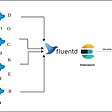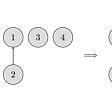# Explanation

• use the diameter of a tree template to solve it
• use the topological sort to solve this one.

# Using diameter of a tree

1. The result at most have two nodes. 1) when the longest path’s length is odd, we have one node as res. 2) when the longest path’s lenght is even, we have two nodes.
2. The result nodes can only exist in the longest path.
3. The longest path is the diameter of the tree
4. The diameter of a tree is a template problem and it can be solved by using two BFSs.
1. Two bfs to find the diameter
2. Use DFS to find the middle points of the longest path from the start node and end node of the longest path.

# C++ code

`class Solution {public:    int node;    void bfs(vector<vector<int>>& graph, int n, int firstnode, int& lastnode, int& longestPathLen){        vector<int> current_level = {firstnode};        longestPathLen = 0;        vector<int> seen(n, 0);        seen[firstnode] = 1;        while (!current_level.empty()){            vector<int> next_level;            for (auto & node: current_level){                for (auto & nei: graph[node]){                    if (seen[nei])continue;                    seen[nei] = 1;                    next_level.push_back(nei);                    lastnode = nei;                }            }            current_level = next_level;            if(!current_level.empty())longestPathLen++;        }    }     void dfs(vector<vector<int>>& graph, int i, int curr, int target_depth, unordered_set<int>&s, vector<int>& seen){                 for (auto & nei: graph[i]){             if (seen[nei] || curr > target_depth) continue;             seen[nei] = 1;             if (curr + 1 == target_depth){                 s.insert(nei);             }            dfs(graph, nei, curr + 1, target_depth, s, seen);         }     }    vector<int> findMinHeightTrees(int n, vector<vector<int>>& edges) {         if (n == 1) return {0};        vector<vector<int>> graph(n + 1);        for (auto & e: edges){            graph[e].push_back(e);            graph[e].push_back(e);        }        // find the diameter of a tree        int firstnode = 0, lastnode = 0, longestPathLen = 0;        bfs(graph, n, firstnode, lastnode, longestPathLen);        firstnode = lastnode;        longestPathLen = 0;        bfs(graph, n, firstnode, lastnode, longestPathLen);                        // find the nodes which has a distance of half of the longest path to the startnode and endnode        // if the path length is odd, we need find half lenght and half length + 1        int depth = longestPathLen / 2;        unordered_set<int> s1, s2;        vector<int> seen(n, 0);        dfs(graph, firstnode, 0, depth, s1, seen);        seen.assign(n, 0);        dfs(graph, lastnode, 0, depth, s2, seen);        if (longestPathLen %2 != 0){            depth ++;             seen.assign(n, 0);            dfs(graph, firstnode, 0, depth, s1, seen);            seen.assign(n, 0);            dfs(graph, lastnode, 0, depth, s2, seen);        }                vector<int> ret;        for (auto& x: s2){            if (s1.find(x) != s1.end()){                ret.push_back(x);            }        }        return ret;            }};`

# C++

`const int N = 2e4 + 10;int indegree[N];// indicate whether the node is explored or notint explored[N];class Solution {    public:    vector<int> findMinHeightTrees(int n, vector<vector<int>>& edges) {        vector<int> ret;        memset(indegree, 0, sizeof indegree);        memset(explored, 0, sizeof explored);        // build the graph        vector<vector<int>> graph(n);        for (auto &e: edges)        {            indegree[e]++;indegree[e]++;            graph[e].push_back(e);            graph[e].push_back(e);        }        // explore the graph by using a topological way.         // Attention, as the graph is bidirectional,        // we should explore the indegree with 1 nodes.        int explored_sofa = 0;        vector<int> current_level;        for (int i = 0; i < n; ++i)             if(indegree[i] == 1)                current_level.push_back(i);        // when the unexploed nodes is 1 or 2, break.        while (n - explored_sofa > 2)        {            vector<int> next_level;            for (auto & node: current_level)            {                explored[node] = 1;                explored_sofa++;                for (auto & nei: graph[node])                {                    if (--indegree[nei] == 1)                        next_level.push_back(nei);                }            }            current_level = next_level;        }        // the unexplored nodes will be our result.        for (int i = 0; i < n; ++i)if(!explored[i])ret.push_back(i);        return ret;}};`

--

--

--

## More from Jimmy Shen

Data Scientist/MLE/SWE @takemobi

Love podcasts or audiobooks? Learn on the go with our new app.

## Docker Log Management Using EFK Stack## Developer Incentives in Sia## Build and run your sample app with Docker (demo)## Elevated access for dotnet commands## Best Practices for Kubernetes Network Policies## The worst abuse of the C preprocessor (Jim Hague 1986)## Jimmy Shen

Data Scientist/MLE/SWE @takemobi

## Union Find Disjoint Set: The most underrated data structure## What actually is “Probabilistic record linkage”